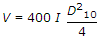# Civil Engineering - Water Resources Engineering

### Exercise :: Water Resources Engineering - Section 1

36.

If the potential infiltration of a water shed having a soil with fair pasture cover, is 10 cm and rainfall is 12 cm, the direct run off is :

 A. 2 cm B. 3 cm C. 5 cm D. 8 cm.

Explanation:

No answer description available for this question. Let us discuss.

37.

The quantity of water retained by the sub-soil against gravity, is known

 A. yield B. porosity C. specific yield D. specific retention E. none of these.

Explanation:

No answer description available for this question. Let us discuss.

38.

Pick up the incorrect statement from the following :

 A. The rate of flow of water through a unit cross-sectional area under a unit hydraulic gradient, is called coefficient of permeability B. The rate of flow of water through a vertical strip of the acquifer of unit width and full depth under a unit hydraulic gradient, is called coefficient of transmissibility C. The flow of water through acquifers, is governed by the Darcy's law D. The term 'transmissibility' was introduced by Meinzer E. The ratio of coefficient of transmissibility and coefficient of permeability, is equal to the depth of acquifer through which water flows.

Explanation:

No answer description available for this question. Let us discuss.

39.

With the usual meanings ofletters, the equationis used for determining the velocity of ground water flow in metres per day. It is known as

 A. Meinzer's formula B. Slichter's formula C. Darcy's formula D. Hazen's formula.

Explanation:

No answer description available for this question. Let us discuss.

40.

If the viscosity of ground water is 1.00, the Slitcher's constant is 400, the effective size of soil particles in acquifer is 0.5 mm and hydraulic gradient is 1 in 80, the velocity of flow is

 A. 0.25 m/day B. 0.50 m/day C. 0.75 m/day D. 1.00 m/day E. 1.25 m/day.